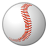# QlikView App Dev

Discussion Board for collaboration related to QlikView App Development.

Announcements
Skip the ticket, Chat with Qlik Support instead for instant assistance.
cancel
Showing results for
Did you mean:Creator II

## Calculated Dimension or Set Analysis in a Straight Table

I have a straight table with 3 fields.  FullName, Purchase and PurchaseAmount.  I need to Sum the Purchase and PurchaseAmount fields.  I then would like to apply a condition on each field before I display the results.  I would only like to see the results where the Sum(Purchase) < 5 AND where the Sum(PurchaseAmount) < 1000.

I have tried using Set Analysis and Calculated Dimensions, but I can't seem to get either way to work.  I've attached some sample data if anyone can please provide a solution.

1 Solution

Accepted SolutionsMaster III

I suggest You Calculated Dimension like

=Aggr(If(Sum(Purchase) < 5 and Sum(PurchaseAmount) < 1000,FullName),FullName)

and check 'Suppress when value is null'.

Regards,

Antonio

2 RepliesMaster III

I suggest You Calculated Dimension like

=Aggr(If(Sum(Purchase) < 5 and Sum(PurchaseAmount) < 1000,FullName),FullName)

and check 'Suppress when value is null'.

Regards,

AntonioMVP

Or this using set analysis:

Dimension:

Name

Expressions:

=Sum({<Name = {"=Sum(Charge) < 5 and Sum(Amount) < 1000"}>}Charge)

=Sum({<Name = {"=Sum(Charge) < 5 and Sum(Amount) < 1000"}>}Amount)Community Browser